# Notes on Miller Indices

The crystal lattice may be regarded as made up of an infinite set of parallel equidistant planes passing through the lattice points which are known as lattice planes. In simple terms, the planes passing through lattice points are called lattice planes’. For a given lattice, the lattice planes can be chosen in a different number of ways.

The orientation of planes or faces in a crystal can be described in terms of their intercepts on the three axes. Miller introduced a system to designate a plane in  a crystal.
Miller introduced a set of three numbers to specify a plane in a crystal. This set of three numbers is known as Miller Indices of the concerned plane. Miller indices is defined as the reciprocals of the intercepts made by the plane on the three axes.

### Procedure for finding Miller Indices

Step 1:  Determine the intercepts of the plane along the axes X,Y and Z in terms of the lattice constants a,b and c.
Step 2:  Determine the reciprocals of these numbers.
Step 3: Find the least common denominator (lcd) and multiply each by this lcd.
Step 4:The result is written in paranthesis.This is called the `Miller Indices’ of the plane in the form (h k l). This is called the `Miller Indices’ of the plane in the form (h k l).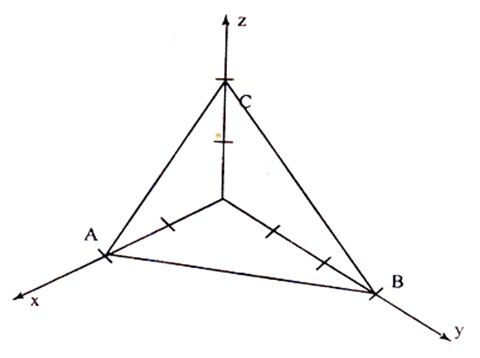Plane ABC has intercepts of 2 units along X-axis,3 units along Y-axis and 2 units along Z-axis

### DETERMINATION  OF ‘MILLER INDICES’

Step 1:The intercepts are 2,3 and 2 on the three axes.

Step 2:The reciprocals are 1/2, 1/3 and 1/2.

Step 3:The least common denominator is ‘6’.
Multiplying each reciprocal by lcd,
we get, 3,2 and 3.

Step 4:Hence Miller indices for the plane ABC is (3 2 3)

### IMPORTANT FEATURES OF MILLER INDICES

For the cubic crystal especially, the important features   of Miller indices are, A plane which is parallel to any one of the co-ordinate axes has an intercept of infinity (¥). Therefore the Miller index for that axis is zero; i.e. for an intercept at infinity, the corresponding index is zero.
ExampleIn the above plane, the intercept along X axis is 1 unit. The plane is parallel to Y and Z axes. So, the intercepts along Y and Z axes are ¥’.

Now the intercepts are 1, ¥ and ¥.
The reciprocals of the intercepts are = 1/1, 1/¥ and 1/¥.
Therefore the Miller indices for the above plane is (1 0 0).

### IMPORTANT FEATURES OF MILLER INDICES

• A plane passing through the origin is defined in terms of aparallel plane having non zero intercepts.
• All equally spaced parallel planes have same ‘Miller indices’ i.e. The Miller indices do not only define a particular plane but also a set of parallel planes. Thus the planes whose intercepts are 1, 1,1; 2,2,2; -3,-3,-3 etc., are all represented by the same set of Miller indices.
• It is only the ratio of the indices which is important in this notation. The (6 2 2) planes are the same as (3 1 1) planes.
•   If a plane cuts an axis on the negative side of the origin,corresponding  index is negative. It is represented by a bar, like  (1* 0 0). i.e. Miller indices (1* 0 0) indicates that the plane  has an intercept in the –ve X –axis.

NOTE: here due to technical reasons bar above a numerical value is shown as 1*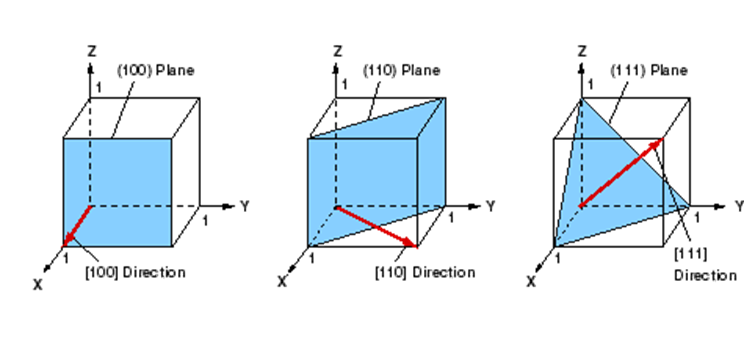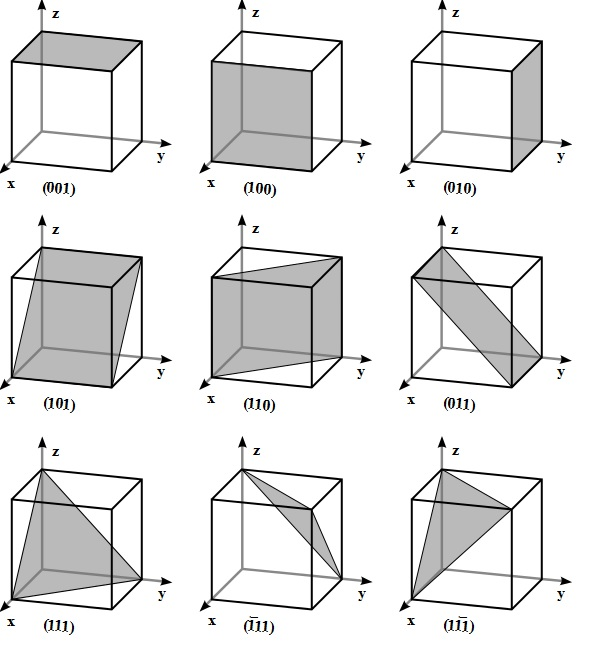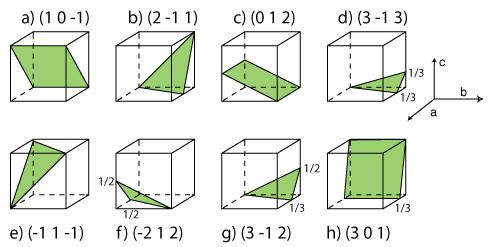• If (h k l) is the Miller indices of a crystal plane then the intercepts made by the plane with the crystallographic axes are given as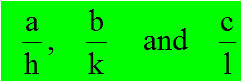1.  A certain crystal has lattice parameters of 4.24, 10 and 3.66 Å on X,   Y, Z axes respectively. Determine the Miller indices of a plane   having intercepts of 2.12, 10 and 1.83 Å on the X, Y and Z axes.
Lattice parameters are = 4.24, 10 and 3.66 Å
The intercepts of the given plane = 2.12, 10 and 1.83 Å
i.e. The intercepts are, 0.5, 1 and 0.5.
Step 1:  The Intercepts are 1/2, 1 and 1/2.
Step 2:  The reciprocals are 2, 1 and 2.
Step 3:  The least common denominator  is 2.
Step 4:  Multiplying the lcd by each reciprocal we get, 4, 2 and 4.
Step 5:  By writing them in parenthesis we get (4 2 4)
Therefore the Miller indices of the given plane is (4 2 4) or (2 1 2).
2.  Calculate the miller indices for the plane with intercepts 2a,   - 3b and 4c               the  along the crystallographic axes.
The intercepts are 2, - 3 and 4
Step 1:  The intercepts are 2, -3 and 4 along the 3 axes
Step 2: The reciprocals are
Step 3: The least  common denominator is 12.
Multiplying each reciprocal by lcd, we get   6 -4 and 3       _
Step 4: Hence the Miller indices for the plane is            (6,4,3)• The angle ‘q’ between any two crystallographic directions [u1 v1 w1] and [u2 v2 w2] can be calculated easily. The angle ‘q’ is  given by,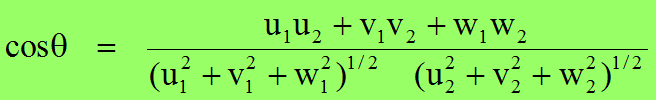• The direction [h k l] is perpendicular to the plane (h k l)

• The relation between the interplanar distance and the interatomic distance is given by,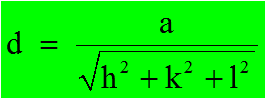• where h, k and l are the miller indices and  a is length of side of cube.Consider a cubic crystal of side ‘a’, and a plane ABC. This plane belongs to a family of planes whose Miller indices are (h k l) because  Miller indices represent a set of planes.

Let ON =d, be the perpendicular distance of the plane A B C from the origin.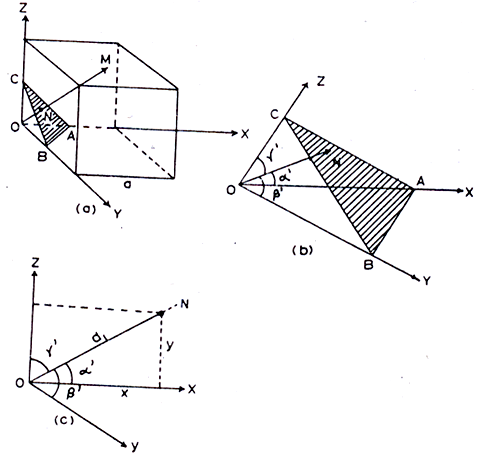the interplanar spacing between two adjacent parallel planes of Miller indices (h k l ) is given by, NM = OM – ON i.e.Interplanar spacing

• ### Example

The lattice constant for a unit cell of aluminum is 4.031Å Calculate the interplanar space of (2 1 1) plane.

a =  4.031 Å
(h k l)  = (2 1 1)
Interplanar spacing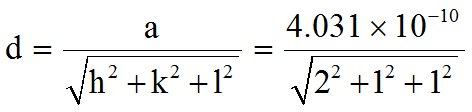d = 1.6456 Å

• ### Example:

Find the perpendicular distance between the two planes indicated by   the Miller indices (1 2 1) and (2 1 2) in a unit cell of a cubic lattice   with a lattice constant parameter ‘a’.
We know  the perpendicular distance between the origin and the plane is (1 2 1)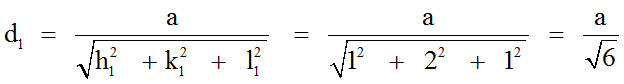and the perpendicular distance between the origin and the plane  (2 1 2),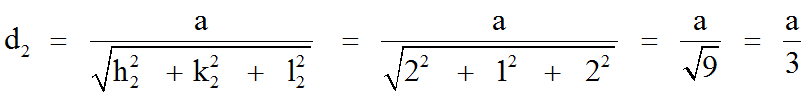The perpendicular distance between the planes (1 2 1) and (2 1 2) are,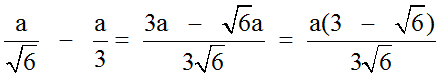d = d1 – d2  =

(or)  d = 0.0749 a.